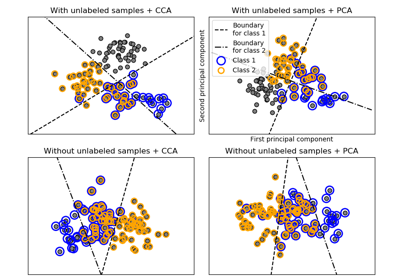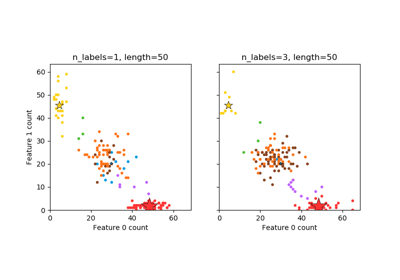# sklearn.datasets.make_multilabel_classification¶

sklearn.datasets.make_multilabel_classification(n_samples=100, n_features=20, n_classes=5, n_labels=2, length=50, allow_unlabeled=True, sparse=False, return_indicator='dense', return_distributions=False, random_state=None)[source]

Generate a random multilabel classification problem.

For each sample, the generative process is:
• pick the number of labels: n ~ Poisson(n_labels)
• n times, choose a class c: c ~ Multinomial(theta)
• pick the document length: k ~ Poisson(length)
• k times, choose a word: w ~ Multinomial(theta_c)

In the above process, rejection sampling is used to make sure that n is never zero or more than n_classes, and that the document length is never zero. Likewise, we reject classes which have already been chosen.

Read more in the User Guide.

Parameters: n_samples : int, optional (default=100) The number of samples. n_features : int, optional (default=20) The total number of features. n_classes : int, optional (default=5) The number of classes of the classification problem. n_labels : int, optional (default=2) The average number of labels per instance. More precisely, the number of labels per sample is drawn from a Poisson distribution with n_labels as its expected value, but samples are bounded (using rejection sampling) by n_classes, and must be nonzero if allow_unlabeled is False. length : int, optional (default=50) The sum of the features (number of words if documents) is drawn from a Poisson distribution with this expected value. allow_unlabeled : bool, optional (default=True) If True, some instances might not belong to any class. sparse : bool, optional (default=False) If True, return a sparse feature matrix New in version 0.17: parameter to allow sparse output. return_indicator : ‘dense’ (default) | ‘sparse’ | False If dense return Y in the dense binary indicator format. If 'sparse' return Y in the sparse binary indicator format. False returns a list of lists of labels. return_distributions : bool, optional (default=False) If True, return the prior class probability and conditional probabilities of features given classes, from which the data was drawn. random_state : int, RandomState instance or None (default) Determines random number generation for dataset creation. Pass an int for reproducible output across multiple function calls. See Glossary. X : array of shape [n_samples, n_features] The generated samples. Y : array or sparse CSR matrix of shape [n_samples, n_classes] The label sets. p_c : array, shape [n_classes] The probability of each class being drawn. Only returned if return_distributions=True. p_w_c : array, shape [n_features, n_classes] The probability of each feature being drawn given each class. Only returned if return_distributions=True.

## Examples using sklearn.datasets.make_multilabel_classification¶Multilabel classificationPlot randomly generated multilabel dataset